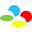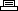Site SearchAnimaniacsPrinter Friendly Version

```Different endings:

The game will display three different endings depending on the
number of script pages that are obtained (less than 10, more
than 10, or all 24).

The password system is based on the following characters (in order):

Y = Yakko
W = Wakko
D = Dot
R = Ralph (guard)
P = Pinky
B = Brain
N = Nurse
C = The CEO.

indicated number of characters. For example, the notation Y + 2
changes Yakko to Dot. Start again at the top of the list if
list. For example, the notation N + 3 changes the Nurse to Wakko.

The password is in a grid format where each position is indicated by
the following:

A1, B1, C1, D1
A2, B2, C2, D2
A3, B3, C3, D3
All levels and all script pages:
Enter CYWD RPBN CYYR as a password.

Create an empty base password by starting a game and dying without
doing anything. One of eight base passwords will be displayed. Edit
Notes section:

Stage completion
Intro: B1+1
Aquatic: A1+1
Fantasy: B1+4
Pinky/Brain: A1+4

Script pages
Aquatic: C2+2, C2+4, B2+2, B2+4, B2+1, C2+1
Fantasy: A2+4, A2+2, D1+1, A2+1
Sci-Fi : D1+2, C1+1, D1+4, C1+2, C1+4
Adventure: A3+1, A3+2, A3+4, D2+4, D2+1, D2+2
Pinky/Brain: B3+2, B3+4, B3+1

Perfect ending

Start from the base code, subtract 1 from all characters
except C3 and D3, add 2 to C3.
```